# Computing the Center of an Area Using Numerical Integration

Problem

You need to integrate a function represented by tabular values to determine the area enclosed by the function and the center of that area.

Solution

Apply a numerical integration technique in your spreadsheet (as discussed in earlier recipes) to compute the area, and take the first moment of the area to compute the centroid.

Discussion

It often happens in science and engineering that you need to compute the area under a curve or the area of some nonprimitive geometric shape. Often the center of that area is of some significance. When working with probability distributions, the area and moments of the area yield important information. In structural mechanics, areas and moments of areas are important for computing stress. Naval architects compute areas and moments of areas to assess how ships float. These are just a few examples of how areas and moments of areas are commonly found in science and engineering.

In this recipe, we'll consider the first moment of an area, which is computed according to the formula: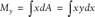This formula yields the first moment of the area about the y-axis. The center of area is computed according to the formula: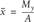In this formula, My is the first moment of the area and A is the area.

Now, let's consider an example. This time, instead of using the trapezoidal rule, I'll use another common integration rule known as Simpson's rule .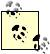There's another Simpson's rule known as Simpson's second rule or the 3/8ths rule.

The general formula for Simpson's rule is: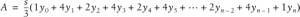This formula is a little different from the formula for the trapezoidal rule. Notice the coefficients in this formula; they follow the pattern 1, 4, 2, 4, 2, 4, 1. The first and last coefficients are always 1, while the middle terms alternate between 4 and 2, with the second and second to last coefficients always 4. Generally, Simpson's rule yields more accurate results than the trapezoidal rule for the same number of samples because Simpson's rule approximates the function, or curve, being integrated using a second-order polynomial as opposed to a linear approximation as is used in the trapezoidal rule. Application of Simpson's rule requires an odd number of samples, ys in the general formula, which makes n an even number. Further, just as in the trapezoidal rule, the spacing, s, between x-values must be uniform.

In this example, we're not dealing with a known analytic function. Instead we have tabular y-values corresponding to a set of x-values. In the real world, these could be measured coordinates from some geometry, or experimentally obtain data, for example.

Figure 10-2 shows the data for this example. x- and y-values are shown in columns B and C, and the coefficients are shown in column D. The little chart on the right shows the shape of the curve represented by this data.

Calculating the area under this curve proceeds in a manner similar to that discussed earlier in Recipe 10.1. Area is computed in cell C19 using the formula =C17/3*SUMPRODUCT(C5:C15,D5:D15). Here again, I use the SUMPRODUCT formula to simultaneously sum the products of the x- and y-values.

The first step in computing the center of the area is to compute the first moment of the area about each axis. This allows you to compute both the x- and y-coordinates of the center of area. To get the coordinates, you have to divide the first moment of the area by the area.

Figure 10-2. Area and area moments example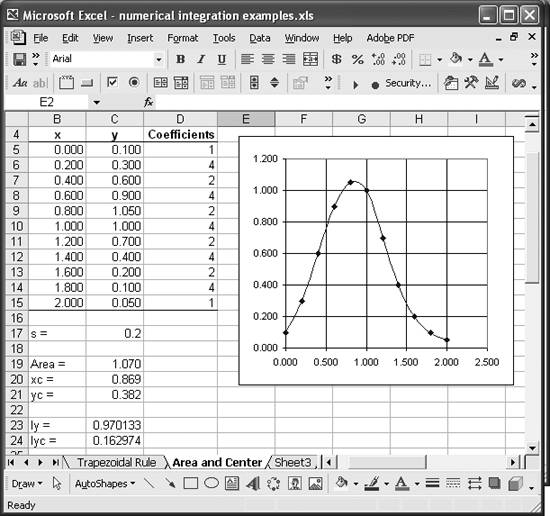The x-coordinate of the center of area is computed in cell C20 using the formula =(C17/3*SUMPRODUCT(B5:B15,C5:C15,D5:D15))/C19. The numerator in this formula computes the first moment of the area about the y-axis using Simpson's rule. This is achieved by again using the SUMPRODUCT formula to compute the sum of the products of x, y, and the coefficients. Dividing by the area, contained in cell C19, yields the x-coordinate to the center of area relative to the origin.

The y-coordinate of the center of area is computed in a similar manner in cell C21 using the formula =(C17/3*SUMPRODUCT(C5:C15,C5:C15/2,D5:D15))/C19. This formula is generally similar to the one used to compute the x-coordinate; however, this time you don't use the x-value at all. To compute the first moment about the x-axis, you multiply the y-value by the distance from the x-axis to the center of the ordinate, which is just half of y. Take a look at the third parameter in the SUMPRODUCT term; it includes a cell range divided by 2. Excel interprets this as an instruction to divide each value in the cell range C5:C15 by 2 before multiplying it by the other values from the other ranges in the SUMPRODUCT function. This is convenient, as it saves having to set up another column of data to compute y/2.You can use other mathematical operators and functions in a similar manner. Clever use of this sort of syntax allows you to construct concise formulas.

Dividing the first moment of the area about the x-axis by the area yields the y-coordinate to the center of area. The result is shown in cell C21 in Figure 10-2.

You can easily extend the example discussed here to compute the second moment of the area. See Recipe 10.4 for more information.Excel Scientific and Engineering Cookbook (Cookbooks (OReilly))
ISBN: 0596008791
EAN: 2147483647
Year: N/A
Pages: 206
Authors: David M Bourg